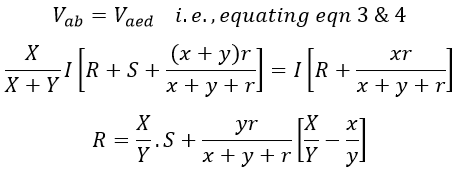# Kelvin's Double Bridge for Measuring Low Resistance - Circuit & Working

The Kelvin bridge or Thompson bridge is one of the methods of measuring the value of unknown resistance less than 1Ω. It is the most accurate method of measuring low resistance compared to other methods. Kelvin's double bridge method is the modified form of the Wheatstone Bridge.

## Need for Kelvin's Double Bridge Circuit :

For the measurement of low resistances, special types of construction and techniques are required. This is because measurement of medium and high resistances involves the resistance of their connecting leads and contacts which does not make any considerable errors in the value.

But, in the case of low resistances, the value of resistance will be less than or equal to 1Ω. Hence, the resistances of leads and contacts cannot be neglected.

Consider an example of measurement of a resistance of 50Ω which involves the connecting leads and contact resistance of 0.025Ω. Hence this measurement involves an error of 0.05%. If the measurement of 0.25Ω is done under similar conditions then the error will be 10% which cannot be neglected under any circumstances.

Hence, it is necessary to make a special type of construction for low resistances which is shown in the figure below.

It consists of 4 terminals in which a, b are to be connected in the circuit for the current flow and c, d to measure the voltage drop across the low resistances. Hence, the lead and contact resistances will not be involved in the measurement.

But, this type of resistor cannot be used for measurements in the ordinary bridge like the Wheatstone Bridge, which measures the value of resistance low or high but not the exact.

Therefore, measurement of low-value resistance using Kelvin's bridge is superior to Wheatstone bridge. The error introduced by the contact/lead resistance is quite large and hence, will give an inaccurate reading. In order to avoid this problem, Kelvin's double bridge is used.

It is so constructed that the contact resistance will not come into the picture while measuring the value of low resistance. Hence, Kelvin's double bridge greatly improves the accuracy of measurement by eliminating the effect of contact resistance.

## Principle and Working of Kelvin's Double Bridge Circuit :

The arrangement of Kelvin's double bridge for the measurement of low-value resistance is shown in the below figure.

Here, R is the unknown resistance to be measured, S is the standard resistance and 'r' is the resistance of the connecting lead. G is the galvanometer and is connected in such a way that the effect of r is eliminated. The circuit incorporates two sets of ratio arms i.e., X, Y, and x, y.

#### Under balanced condition,Vab = VacdFrom figure,Substituting equation 2 in 1, we get,Under balanced condition,But,Hence, the value of unknown resistance R is independent of the contact resistance 'r' even though it is present in the circuit. This is possible only if the two sets of ratio arms have equal values.

Thus by introducing another set of ratio arms (i.e., xy) into the bridge, the effect of contact resistance and resistance of the connecting leads can be eliminated.

Do not enter any spam links and messages